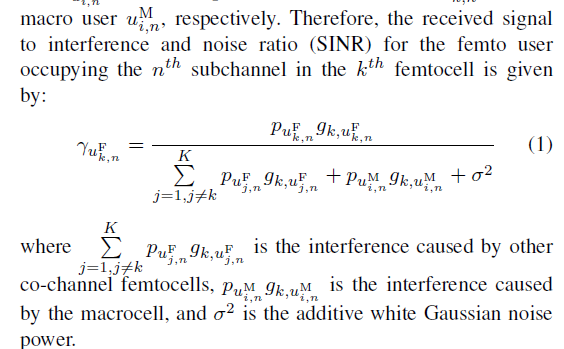# Expressing shannon capacity in CVX

I am wondering why CVX is reporting error in line 6 of the codes below which I have written to maximize the capacity of a femtocell user in an heterogeneous cellular network.

Shannon’s Capacity is C = B.*log(1+SINR).

The concern is in the expression for the SINR part. The equation for SINR is shown in the diagram. I will appreciate any assitance whatsoever.
Thanks

cvx_begin
variables pf(MD,FAP) pm(MD,FAP)
expression Cap_femto_obj
for u = 1:MD
for v = 1:FAP
Cap_femto_obj = Cap_femto_obj + B_RB.*((entr(1+(times( (pf.*Gd_fu), (sigma2 + pf.*G_co + pm.*G_cross), ‘./’ ))))./entr(2));
end
end
objective = Cap_femto_obj
maximize(objective)
subject to
0<=pf; pf<=(10^(23/10))/1000;
cvx_endWhat does the FAQ say about the specific error you’re getting? (And what is that error?)

The specific error I am getting is:

Error using .* (line 173)
Disciplined convex programming error:
Cannot perform the operation: {real affine} ./ {real affine}

Error in ./ (line 19)
z = times( x, y, ‘./’ );

Error in line 6
Cap_femto_obj = Cap_femto_obj + B_RB.*((entr(1+((pf.*Gd_fu)./(sigma2
+ pf.*G_co +pm.*G_cross))))./entr(2));

The problem is a convex optimization problem, the related FAQ section states that “a convex, concave, or affine function may accept an affine expression (of compatible size) as an argument. The result is convex, concave, or affine, respectively.”

This left me wondering why I’m getting the {real affine} ./ {real affine}

The DCP ruleset, referred to in the docs, does not allow you to divide one affine expression by another. And that’s exactly what you’re trying to do with `(pf.*Gd_fu)./(sigma2 + pf.*G_co +pm.*G_cross)`.

CVX is likely not going to be able to solve your problem.

Thanks sir for the response. I have tried to avoid the DCP afine error by convexifying the expression

cvx_begin
variables pf(MD,1) pm(MD,1)
expression Cap_femto_obj
for u = 1:MD
for v = 1:FAP
Cap_femto_obj = Cap_femto_obj + B_RB.*((log(pf)) + (log(Gd_fu)) - log(((sum(exp((log(G_co))+(log(pf))))) +(sum(exp((log(G_cross))+(log(pm)))))+ exp(log(sigma2)))));
end
end
objective = Cap_femto_obj
maximize(objective)
subject to
0<=pf; pf<=(10^(23/10))/1000;
cvx_end

But I am getting another error message

Error using + (line 45)
Matrix dimensions must agree.

Error in CoalitionalGame (line 74)
Cap_femto_obj = Cap_femto_obj + B_RB.*((log(pf)) + (log(Gd_fu)) -
log(((exp((log(G_co))+(log(pf)))) +(exp((log(G_cross))+(log(pm))))+
exp(log(sigma2)))));